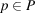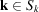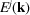International
Tables for
Crystallography
Volume D
Physical properties of crystals
Edited by A. Authier

International Tables for Crystallography (2006). Vol. D, ch. 2.2, p. 298

## Section 2.2.7. Thevector and the Brillouin zone

K. Schwarza*

aInstitut für Materialchemie, Technische Universität Wien, Getreidemarkt 9/165-TC, A-1060 Vienna, Austria
Correspondence e-mail: kschwarz@theochem.tuwein.ac.at

### 2.2.7. Thevector and the Brillouin zone

| top | pdf |

#### 2.2.7.1. Various aspects of thevector

| top | pdf |

Thevector plays a fundamental role in the electronic structure of a solid. In the above, several interpretations have been given for thevector that

 (a) is given in reciprocal space, (b) can be restricted to the first Brillouin zone, (c) is the quantum number for the electronic states in a solid, (d) is quantized due to the periodic boundary conditions, (e) labels the irreducible representation of the lattice translation subgroup T (see Section 2.2.4.3) (f) is related to the momentum [according to (2.2.5.5)] in the free-electron case and (g) is the propagation vector (wavevector) associated with the plane-wave part of the wavefunction (see Fig. 2.2.5.1).

#### 2.2.7.2. The Brillouin zone (BZ)

| top | pdf |

Starting with one of the 14 Bravais lattices, one can define the reciprocal lattice [according to (2.2.2.4)] by the Wigner–Seitz construction as discussed in Section 2.2.2.2. The advantage of using the BZ instead of the parallelepiped spanned by the three unit vectors is its symmetry. Let us take a simple example first, namely an element (say copper) that crystallizes in the face-centred-cubic (f.c.c.) structure. With (2.2.2.4)we easily find that the reciprocal lattice is body-centred-cubic (bcc) and the corresponding BZ is shown in Fig. 2.2.7.1. In this case, f.c.c. Cu hassymmetry with 48 symmetry operations(point group). The energy eigenvalues within a star of(i.e.) are the same, and therefore it is sufficient to calculate one member in the star. Consequently, it is enough to consider the irreducible wedge of the BZ (called the IBZ). In the present example, this corresponds to 1/48th of the BZ shown in Fig. 2.2.7.1. To count the number of states in the BZ, one counts eachpoint in the IBZ with a proper weightto represent the star of thisvector.Figure 2.2.7.1 | top | pdf |The Brillouin zone (BZ) and the irreducible wedge of the BZ for the f.c.c. direct lattice. After the corresponding figure from the Bilbao Crystallographic Server (http://www.cryst.ehu.es/ ). The IBZ for any space group can be obtained by using the option KVEC and specifying the space group (in this case No. 225).

#### 2.2.7.3. The symmetry of the Brillouin zone

| top | pdf |

The BZ is purely constructed from the reciprocal lattice and thus only follows from the translational symmetry (of the 14 Bravais lattices). However, the energy bands, withlying within the first BZ, possess a symmetry associated with one of the 230 space groups. Therefore one can not simply use the geometrical symmetry of the BZ to find its irreducible wedge, although this is tempting. Since the effort of computing energy eigenvalues increases with the number ofpoints, one wishes to restrict such calculations to the basic domain, but the latter can only be found by considering the space group of the corresponding crystal (including the basis with all atomic positions).

One possible procedure for finding the IBZ is the following. First a uniform grid in reciprocal space is generated by dividing the three unit-cell vectorsby an integer number of times. This is easy to do in the parallelepiped, spanned by the three unit-cell vectors, and yields a (more-or-less) uniform grid ofpoints. Now one must go through the complete grid ofpoints and extract a list of non-equivalentpoints by applying to eachpoint in the grid the point-group operations. If apoint is found that is already in the list, its weight is increased by 1, otherwise it is added to the list. This procedure can easily be programmed and is often used whenintegrations are needed. The disadvantage of this scheme is that the generatedpoints in the IBZ are not necessarily in a connected region of the BZ, since one member of the star ofis chosen arbitrarily, namely the first that is found by going through the complete list.